# What is Logarithmic Time Complexity? A Complete Tutorial

Algorithms are extremely important in computer programming because a whole computer model runs when several algorithms work together. Choosing an efficient algorithm can be a tough choice to make for this complex analysis of the algorithm. There is various order of time complexities for the determination of algorithm out of which some are most efficient and some are worst. So, we have to take care of this complexity for the better performance of any program. In this blog, we will look in-depth into the Logarithmic Complexity. We will also do various comparisons between different logarithmic complexities, when and where such logarithmic complexities are used, several examples of logarithmic complexities, and much more. So let’s get started.What is Logarithmic Time Complexity

## What is meant by Complexity Analysis?

The primary motive to use DSA is to solve a problem effectively and efficiently. How can you decide if a program written by you is efficient or not? This is measured by complexities. Complexity is of two types:

### What is Space Complexity?

The space Complexity of an algorithm is the space taken by an algorithm to run the program for a given input size. The program has some space requirements necessary for its execution these include auxiliary space and input space. The important standard for comparison of algorithms is the space taken by the algorithm to run for a given input size Hence it needs to be optimized.

### What is Time Complexity?

In Computer science, there are various problems and several ways to solve each of these problems using different algorithms. These algorithms may have varied approaches, some might be too complex to Implement while some may solve the problem in a lot simpler way than others. It is hard to select a suitable and efficient algorithm out of all that are available. To make the selection of the best algorithm easy, calculation of complexity and time consumption of an algorithm is important this is why time complexity analysis is important, for this asymptotic analysis of the algorithm is done.

There are three cases denoted by three different notations of analysis:

### How to measure complexities?

Below are some major order of Complexities are:

• Constant: If the algorithm runs for the same amount of time every time irrespective of the input size. It is said to exhibit constant time complexity.
• Linear: If the algorithm runtime is linearly proportional to the input size then the algorithm is said to exhibit linear time complexity.
• Exponential: If the algorithm runtime depends on the input value raised to an exponent then it is said to exhibit exponential time complexity.
• Logarithmic: When the algorithm runtime increases very slowly compared to an increase in input size i.e. logarithm of input size then the algorithm is said to exhibit logarithmic time complexity.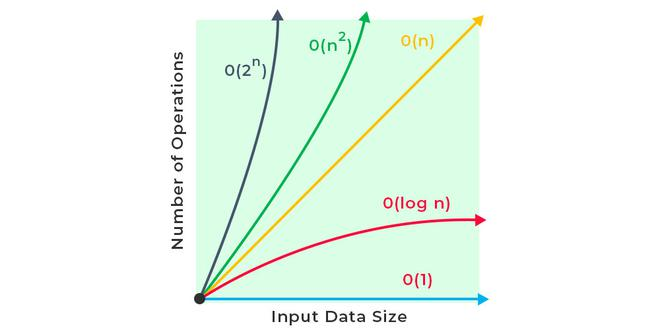Measurement of Complexity analysis

## What is a logarithm?

The power to which a base needs to be raised to reach a given number is called the logarithm of that number for the respective base.
For finding logarithmic two necessary factors that need to be known are base and number.Examples:

logarithm of 8 for base 2 = log2(8) = 3,
Explanation: 23 = 8 Since 2 needs to be raised to a power of 3 to give 8, Thus logarithm of 8 base 2 is 3.

logarithm of 81 for base 9 = log9(81) = 2,
Explanation: 92 = 81 Since 9 needs to be raised to a power of 2 to give 81, Thus logarithm of 81 base 9 is 2.

Note: An exponential function is the exact opposite of a logarithmic function. When a value is being multiplied repeatedly it is said to grow exponentially whereas when the value is being divided repeatedly it is said to grow logarithmically.

## Different types of Logarithmic Complexities

Now that we know what is a logarithm, let’s deep dive into different types of logarithmic complexities that exists, such as:

## 1. Simple Log Complexity (Loga b)

Simple logarithmic complexity refers to log of b to the base a. As mentioned, it refers to the time complexity in terms of base a. In design and analysis of algorithms, we generally use 2 as the base for log time complexities. The below graph shows how the simple log complexity behaves.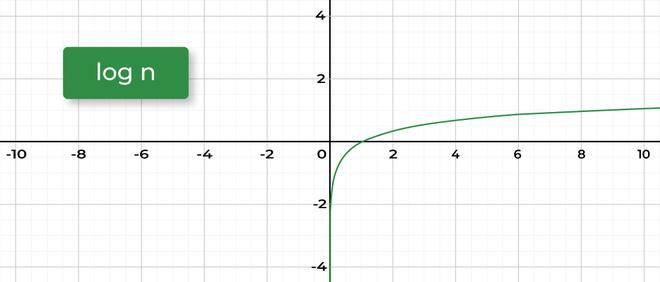Simple Log Complexity (Log(a) b)

There are several standard algorithms that have logarithmic time complexity:

## 2. Double Logarithm (log log N)

Double logarithm is the power to which a base must be raised to reach a value x such that when the base is raised to a power x it reaches a value equal to given number.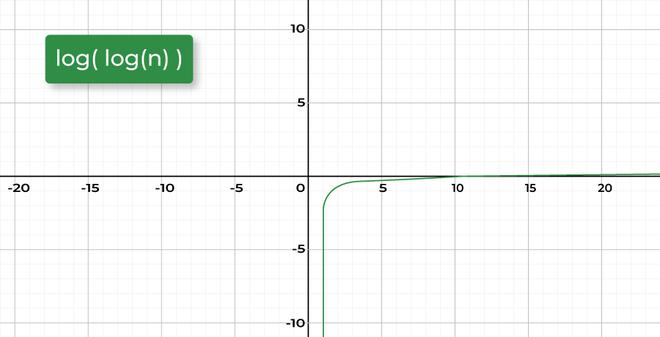Double Logarithm (log log N)

Example:

logarithm (logarithm (256)) for base 2 = log2(log2(256)) = log2(8) = 3.

Explanation: 28 = 256, Since 2 needs to be raised to a power of 8 to give 256, Thus logarithm of 256 base 2 is 8. Now 2 needs to be raised to a power of 3 to give 8 so log2(8) = 3.

## 3. N logarithm N (N * log N)

N*logN complexity refers to product of N and log of N to the base 2. N * log N time complexity is generally seen in sorting algorithms like Quick sort, Merge Sort, Heap sort. Here N is the size of data structure (array) to be sorted and log N is the average number of comparisons needed to place a value at its right place in the sorted array.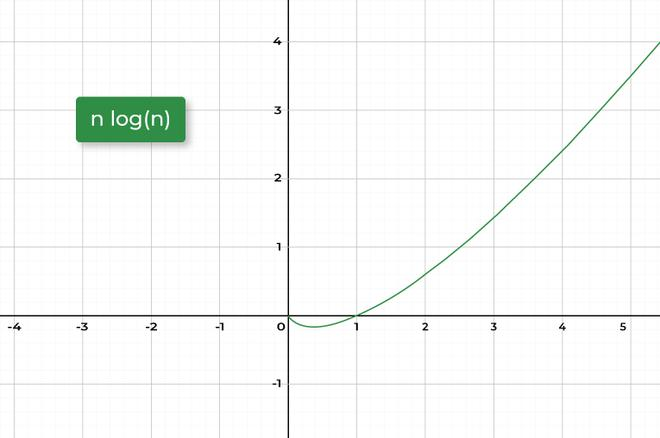N * log N

## 4. logarithm2 N (log2 N)

log2 N complexity refers to square of log of N to the base 2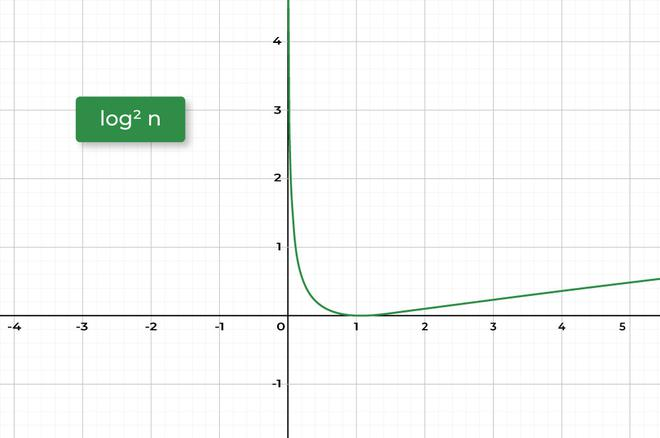log2 N

## 5. N2 logarithm N (N2 * log N)

N2*log N complexity refers to product of square of N and log of N to the base 2. This Order of time complexity can be seen in case where an N * N * N 3D matrix needs to be sorted along the rows. The complexity of sorting each row would be N log N and for N rows it will be N * (N * log N). Thus the complexity will be N2 log N,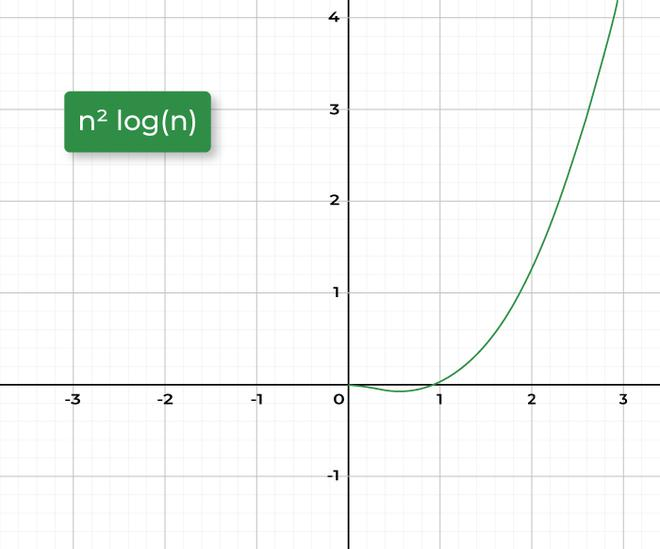N2 * log N

## 6. N3 logarithm N (N3 log N)

N3*log N complexity refers to product of cube of N and log of N to the base 2. This Order of time complexity can be seen in cases where an N * N matrix needs to be sorted along the rows. The complexity of sorting each row would be N log N and for N rows it will be N * (N * log N) and for N width it will be N * N * (N log N). Thus the complexity will be N3 log N,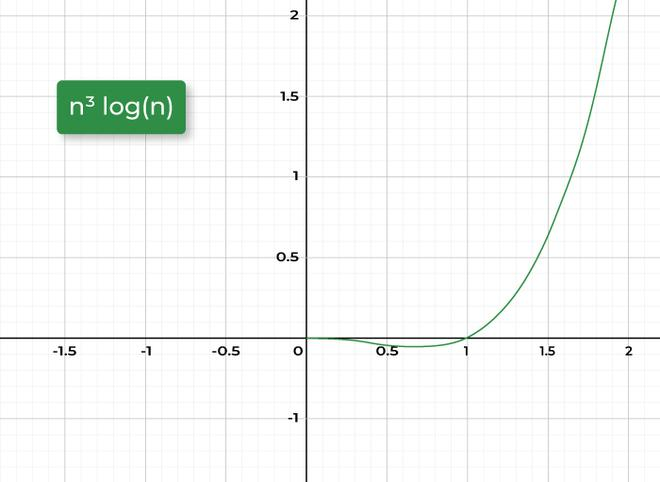N3 log N

## 7. logarithm √N (log √N)

log √N complexity refers to log of square root of N to the base 2.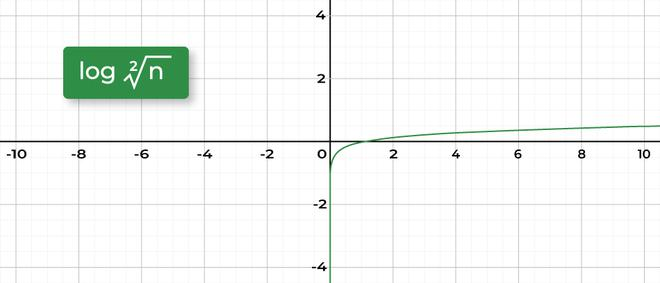log √N

## Examples To Demonstrate Logarithmic Time Complexity

### Example 1: loga b

Task: We have a number N which has an initial value of 16 and the task is to reduce the given number to 1 by repeated division of 2.
Approach:

• Initialize a variable number_of_operation with a value 0 .
• Run a for loop from N till 1.
• In each iteration reduce the value of N to half.
• Increment the number_of_operation variable by one.
• Return the number_of_operation variable.

Implementation:

## C++

 `#include ` `using` `namespace` `std;` ` `  `int` `main()` ` `  `    ``int` `N = 16;` `    ``int` `number_of_operations = 0;` ` `  `    ``cout << ``"Logarithmic reduction of N: "``;` `    ``for` `(``int` `i = N; i > 1; i = i / 2) ` `        ``cout << i << ``" "``;` `        ``number_of_operations++;` `    ` `    ``cout << ``'\n'` `         ``<< ``"Algorithm Runtime for reducing N to 1: "` `         ``<< number_of_operations;`

## Javascript

 `let number_of_operations = 0;` ` `  `for``(let i=n; i>=1; i=i/2) ` `   ``console.log(i);` `   ``number_of_operations++;` ` `  `console.log(number_of_operations);`
Output

```Logarithmic reduction of N: 16 8 4 2
Algorithm Runtime for reducing N to 1: 4```

#### Explanation:

It is clear from the above algorithm that in each iteration the value is divided by a factor of 2 starting from 16 till it reaches 1, it takes 4 operations.

As the input value gets reduced by a factor of 2, In mathematical terms the number of operations required in this case is log2(N), i.e. log2(16) = 4.
So, in terms of time complexity, the above algorithm takes logarithmic runtime to complete i.e.  log2(N)

### Example 2: Binary search algorithm (log N)

Linearly Searching a value in an array of size N can be very hectic, even when the array is sorted but using binary search this can be done in a lot easier way and in lesser time as the algorithm reduces the search space by half in each operation thus gives a complexity of log2(N), Here base is 2 because process repeatedly reduces to half.

Consider an array Arr[] = 2, 4, 6, 8, 10, 12, 14, 16, 18, If it is required to find the index of 8 then the algorithm will work as following:

## C++

 ` `  `#include ` `using` `namespace` `std;` ` `  `int` `find_position(``int` `val, ``int` `Arr[], ``int` `n, ``int``& steps)` `    ``int` `l = 0, r = n - 1;` ` `  `    ``while` `(l <= r) ` `        ``steps++;` `        ``int` `m = l + (r - l) / 2;` `        ``if` `(Arr[m] == val)` `            ``return` `m;` `        ``else` `if` `(Arr[m] < val)` `            ``l = m + 1;` `        ``else` `            ``r = m - 1;` `    ` `    ``return` `-1;` ` `  `int` `main()` ` `  `    ``int` `Arr = 2, 4, 6, 8, 10, 12, 14, 16 ;` `    ``int` `steps = 0;` ` `  `    ` `    ``int` `idx = find_position(8, Arr, 8, steps);` `    ``cout << ``"8 was present on index: "``<
Output

```8 was present on index: 3
Algorithm Runtime: 1```

#### Explanation:

Binary search works on Divide and conquer approach, In above example In worst case 3 comparisons will be needed to find any value in array. Also the value of log (N) where N is input size i.e. 8 for above example will be 3. Hence the algorithm can be said to exhibit logarithmic time complexity.

### Example 3: Binary search algorithm (log log N)

An example where the time complexity of algorithm is Double logarithmic along with a length factor N is when prime numbers from 1 to N need to be found.

## C++

 `#include ` `using` `namespace` `std;` `const` `long` `long` `MAX_SIZE = 1000001;` ` `  `vector<``long` `long``> isprime(MAX_SIZE, ``true``);` `vector<``long` `long``> prime;` `vector<``long` `long``> SPF(MAX_SIZE);` ` `  `void` `manipulated_seive(``int` `N)` `    ` `    ``isprime = isprime = ``false``;` ` `  `    ` `    ``for` `(``long` `long` `int` `i = 2; i < N; i++) ` `        ` `        ` `        ``if` `(isprime[i]) ` `            ` `            ``prime.push_back(i);` ` `  `            ` `            ` `            ``SPF[i] = i;` `        ` ` `  `        ` `        ` `        ` `        ` `        ` `        ` `        ` `        ``for` `(``long` `long` `int` `j = 0;` `             ``j < (``int``)prime.size() && i * prime[j] < N` `             ``&& prime[j] <= SPF[i];` `             ``j++) ` `            ``isprime[i * prime[j]] = ``false``;` ` `  `            ` `            ``SPF[i * prime[j]] = prime[j];` `        ` `    ` ` `  `int` `main()` `    ``int` `N = 13; ` ` `  `    ``manipulated_seive(N);` ` `  `    ` `    ``for` `(``int` `i = 0; i < prime.size() && prime[i] <= N; i++)` `        ``cout << prime[i] << ``" "``;` ` `  `    ``return` `0;`

In above example the complexity of finding prime numbers in a range of 0 to N is O(N * log (log (N))).